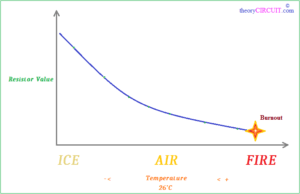# Resistor exposed on different Temperature

Just a Experiment to observe the Resistance value of a normal carbon through hole Resistor when it feels different temperature ranges.

Here a 560Ω, 1/2 watt Resistor is taken as a example and it is exposed on Air (normal room temperature air), ICE and Fire then the value changes of Resistance is observed through digital multimeter.

When putting the observed value of Resistance on a graph we get,Result of this experiment, when the Resistor in room Air temperature there is no change in Resistance value, when exposed on Ice the Resistance value increase greater than actual stated value of Resistor.

When exposed on Fire the Resistance value decreases below the actual value of Resistor.